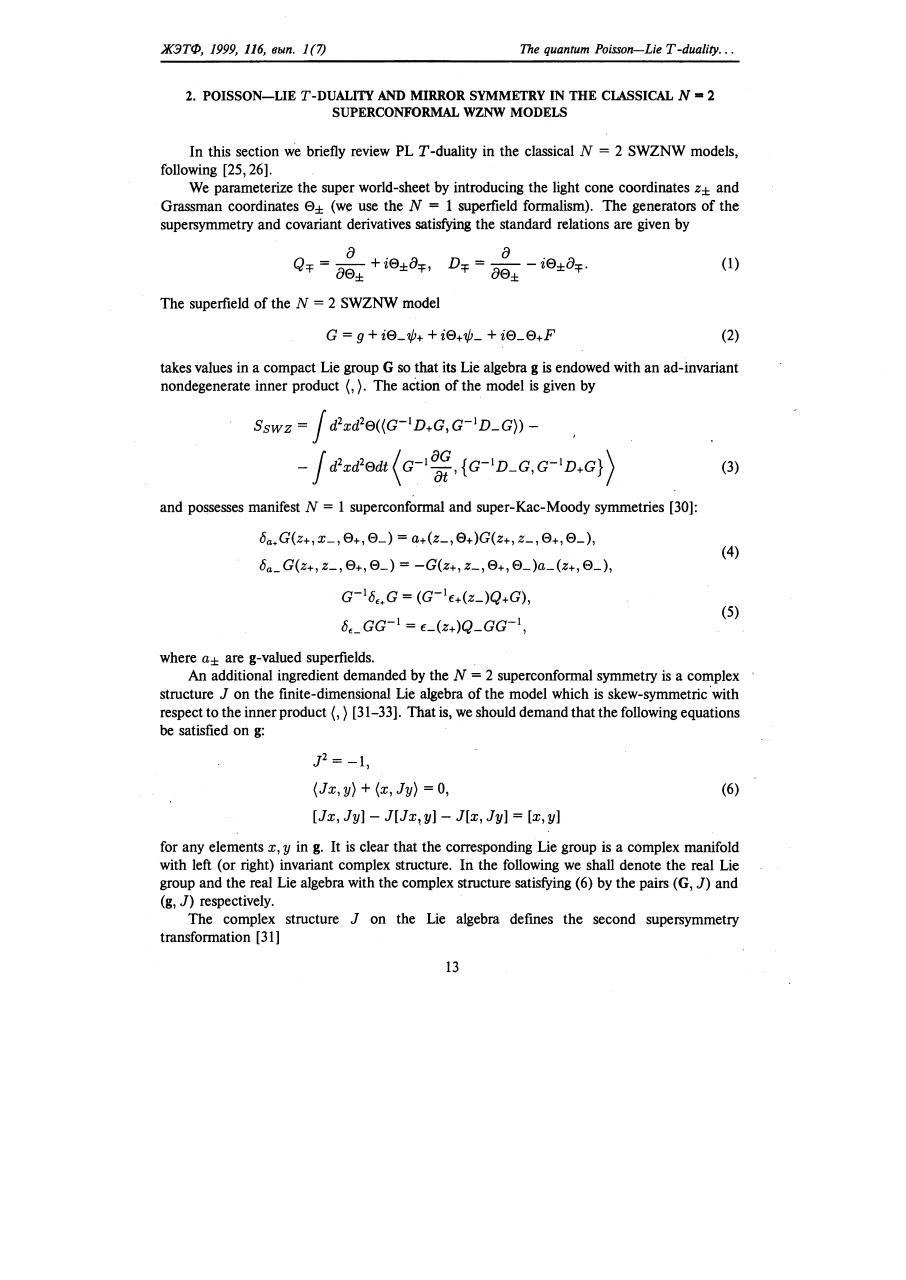# r 116 0011.pdfPage 1 2 34515

#### Text preview

ЖЭТФ,

1999, 116,

выn.

2. POISSON-LIE

Тhe

1 (7)

T-DUALIТY

quantum Poisson-Lie

Т -dua/ity . ..

AND MIRROR SYMMETRY IN ТНЕ CLASSICAL N - 2
WZNW MODELS

SUPERCONFORМAL

In this section we briefly review PL Т -duality in the classical N = 2 SWZNW models,
following [25,26].
We pammeterize the super world-sheet Ьу introducing the light сопе coordinates z± and
Grassman coordinates 0± (we use the N = 1 superfield formalism). The genemtors of the
supersymmetry and covariant derivatives satisfying the standard relations are given Ьу
(1)
Тhe

superfield of the N

= 2 SWZNW model
(2)

takes values in а compact Lie group G so that i18 Lie algebm g 18 endowed with ап ad-invariant
nondegenerate inner product (,). The action of the model is given Ьу

(3)
and possesses manifest N

= 1 superconformal and super-Кac-Moody symmetries :

8a.G(z+, х_, 0+, 0_) = a+(z_, 0+)G(z+, z_, 0+, 0_),
8 а _ G(z+,

z_, 0+, 0_)

= -G(z+, z_, 0+, 0_)a_(z+, 0_),

(4)

G- 18,.G = (G-l€+(Z_)Q+G),
8,_аа- 1

= c(z+)Q_GG- 1,

(5)

where а± are g-valued superfields.
An additional ingredient demanded Ьу the N = 2 superconformal symmetry is а complex
structure J оп the finite-dimensional Lie algebra of the model which is skew-symmetric 'with
respect to the inner product (, ) [31-33]. That is, we should demand that the following equations
ье satisfied оп g:

J2

= -1,

(Jx, у)

+ (х, Jy)

= О,

[Jx, Jy] - J[Jx! у] - J[x, Jy]

(6)

= [х, у]

for апу elements х, у in g. It is clear that the corresponding Lie group is а complex manifold
with left (or right) invariant complex structure. In the following we shall denote the real Lie
group and the real Lie algebra with the complex structure satisfying (6) Ьу the раш (G, J) and
(g, J) respectively.
The complex structure J оп the Lie algebm defmes the second supersymmetry
transformation 
13